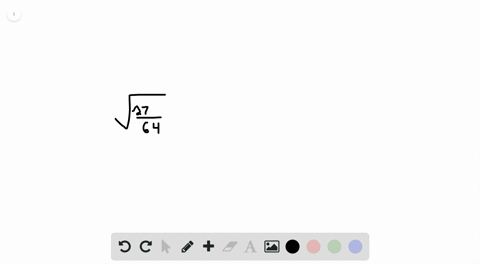Sign up for our free STEM online summer camps starting June 1st!View Summer Courses### Simplify each expression. $$\sqrt{\frac{27}{64}}… 00:40 Problem 14Hofstra University Need more help? Fill out this quick form to get professional live tutoring. Get live tutoring Problem 11 Simplify each expression.$$\sqrt{98}

7$\sqrt{2}$

## Discussion

You must be signed in to discuss.

## Video Transcript

to simplify this expression, we first have to see if there is a squared of 98. So if we think about it, or if we plug it into our calculator, is there a number that when you multiply it by itself, it equals 98? And if you try a few numbers, you find that you don't get the whole number, so we don't want to leave. Her answer is a decimal, so the next thing we have to do is see Well, is there a perfect square that can divide 98? Now? A perfect square is a number that when you multiply it by itself, you get a number. So perfect squares would be 14 nine, 16 25 36 et cetera. One times 12 times 23 times 34 terms for etcetera. So we're trying to see if there's a square root of 90 of 98 or if there's a perfect square that can divide 90 eat. So again you can kind of guess in check. You can start doing 98 divided by start with four or 98 divided by nine were 16 25 until you get to numbers at work. So if you end up doing 98 divided by 49 you get two. And 49 is a perfect square so we can break this up into square root of nine. So I had the squared of, uh, 49 times the square root of two, because 49 times two is 98. Now what happens is that since squared of 49 can simplify square to 49 7 we get seven times the square to two, which is just seven Brad, too. So that would be our final simplified answer without using decimals.